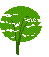Re: Cross-referencing

From: Matthias Clasen <mclasen@sun2.mathematik.uni-freiburg.de>
Date: Sat, 29 Mar 1997 17:24:50 +0100
```Sorry, I just posted this under a wrong subject:

Thanks a lot,

Tony Grahams style-sheet does exactly what I wanted - this list might

Let me try to learn a bit more by making my first question a bit more
complicated. I would like the document (using the sample DTD posted by
Tony

<test>
<theorem id="theorem1">The earth is flat</theorem>
<theorem id="theorem2">The stork didn't really bring me</theorem>
<proof of="theorem2">If the stork brought me, I wouldn't need this
belly button</proof>
<proof of="theorem1">Someone told me</proof>
</test>

To be formatted roughly like this:

Theorem 1: The earth is flat

Theorem 2: The stork didn't really bring me

Proof: If the stork brought me, I wouldn't need this
belly button

Proof of 1: Someone told me

I.e. I want the `of ' part in proof to be omitted, if it is immediately
following the theorem. So the task is to determine if the node
immediately
before the `proof' element is the one the `of' attribute is referring
to.
If I understood the semantics of the ipreced function in 10.2.3
correctly,
the following modification of the proof-related part of Tony Grahams
style-sheet should do what I want:

(element PROOF
(make paragraph
(make sequence
(if (equal? (attribute-string "OF")
(attribute-string  "ID" (ipreced)))
; proof directly following theorem, omit number
(literal "Proof:" )
; proof not directly following theorem
(with-mode theorem
(process-element-with-id
(attribute-string "OF"))))
(process-children))))

But I can't try this as Jade does not understand `ipreced'. This leads
to some questions:

1) Is my idea correct ?

2) Is there a way to do it within the current limitations of Jade ?

Latex-style called varioref which allows `intelligent pagereferences'
which print out
text like `on this page', `on the next page', `on the previous page'.
It
might even be aware if the reference and the referent are on one
or not.

How would one handle things like that in DSSSL ? Is this possible at
all
(since the page-breaks depend on the formatting algorithms which are
not
part of DSSSL) ?

Looking forward for more enlightenment,

Matthias

```